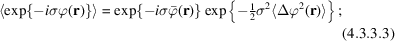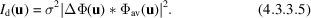International
Tables for
Crystallography
Volume B
Reciprocal space
Edited by U. Shmueli

International Tables for Crystallography (2010). Vol. B, ch. 4.3, p. 542   | 1 | 2 |

Section 4.3.3. Kinematical and pseudo-kinematical scattering

J. M. Cowleya and J. K. Gjønnesb

aArizona State University, Box 871504, Department of Physics and Astronomy, Tempe, AZ 85287–1504, USA, and  bInstitute of Physics, University of Oslo, PO Box 1048, N-0316 Oslo 3, Norway

4.3.3. Kinematical and pseudo-kinematical scattering

| top | pdf |

Kinematical expressions for TDS or defect and disorder scattering according to equation (4.3.1.3)can be obtained by inserting the appropriate atomic scattering factors in place of the X-ray scattering factors in Chapter 4.1.The complications introduced by dynamical diffraction are considerable (see Section 4.3.4). In the most general case, a complete specification of the disordered structure may be needed. However, for thin specimens, approximate treatments of the deviations from kinematical scattering may lead to relatively simple forms. Two such cases are treated in this section, both relying on the small-angle nature of electron scattering. The first is based upon the phase-object approximation, which applies to small angles and thin specimens.

The amplitude at the exit surface of a specimen can always be written as a sum of a periodic and a nonperiodic part, and may in analogy with the kinematical case [equation (4.3.1.1)] be writtenwhere r is a vector in two dimensions. The intensities can be separated in the same way [cf. equation (4.3.1.3)].

When the phase-object approximation applies [Section 2.5.2.4(b)]Then the Bragg reflections are given by Fourier transform of the periodic part, viz:note that an absorption function is introduced.

The diffuse scattering derives fromso thatThus, the kinematical diffuse-scattering amplitude is convoluted with the amplitude function for the average structure, i.e. the set of sharp Bragg beams. When the direct beam,, is relatively strong, the kinematical diffuse scattering will be modified to only a limited extent by convolution with the Bragg reflections. To the extent that the diffuse scattering is periodic in reciprocal space, the effect will be to modify the intensity by a slowly varying function. Thus the shapes of local diffuse maxima will not be greatly affected.

The electron-microscope image contrast derived from the diffuse scattering will be obtained by inserting equation (4.3.3.4)in the appropriate intensity expressions of Section 4.3.8of IT C (2004).

Another approach may be used for extended crystal defects in thin films, e.g. faults normal or near-normal to the film surface. Often, an average periodic structure may not readily be defined, as in the case of a set of incommensurate stacking faults. Kinematically, the projection of the structure in the simplest case may be described by convoluting the projection of a unit-cell structure with a nonperiodic set of delta functions which constitute a distribution function:Then the diffraction-pattern intensity isHere,is the scattering amplitude of the unit whereas the function, where, gives the configuration of spots, streaks or other diffraction maxima corresponding to the faulted structure (see e.g. Marks, 1985).

In the projection (column) approximation to dynamical scattering, the wavefunction at the exit surface may be given by an expression identical to (4.3.3.6), but with a wavefunction,, for the unit in place of the projected potential,.

An intensity expression of the same form as (4.3.3.7)then applies, with a dynamical scattering amplitudefor the scattering unit substituted for the kinematical amplitude.which in the simplest case describes a diffraction pattern with the same features as in the kinematical case. Note thatmay have different symmetries when the incident beam is tilted away from a zone axis, leading to diffuse streaks etc. appearing also in positions where the kinematical diffuse scattering is zero. More complicated cases have been considered by Cowley (1976a) who applied this type of analysis to the case of nonperiodic faulting in magnesium fluorogermanate (Cowley, 1976b).

References

International Tables for Crystallography (2004). Vol. C. Mathematical, Physical and Chemical Tables, edited by E. Prince. Dordrecht: Kluwer Academic Publishers.
Cowley, J. M. (1976a). Diffraction by crystals with planar faults. I. General theory. Acta Cryst. A32, 83–87.
Cowley, J. M. (1976b). Diffraction by crystals with planar faults. II. Magnesium fluorogermanate. Acta Cryst. A32, 88–91.
Marks, L. D. (1985). Image localization. Ultramicroscopy, 18, 33–38.